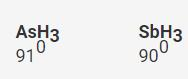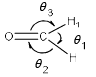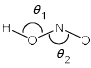Courses

# Test: Chemical Bonding- 3

## 30 Questions MCQ Test Inorganic Chemistry | Test: Chemical Bonding- 3

Description
This mock test of Test: Chemical Bonding- 3 for Chemistry helps you for every Chemistry entrance exam. This contains 30 Multiple Choice Questions for Chemistry Test: Chemical Bonding- 3 (mcq) to study with solutions a complete question bank. The solved questions answers in this Test: Chemical Bonding- 3 quiz give you a good mix of easy questions and tough questions. Chemistry students definitely take this Test: Chemical Bonding- 3 exercise for a better result in the exam. You can find other Test: Chemical Bonding- 3 extra questions, long questions & short questions for Chemistry on EduRev as well by searching above.
QUESTION: 1

Solution:
QUESTION: 2

Solution:
QUESTION: 3

### The correct non-linear and iso-structural pair is:

Solution:

In B the central atoms are S and I+ respectively, having 6 e- each and the others are from G-VII. Hence, they will have the same lone pair repulsion.

Hence B is correct.

QUESTION: 4

Among the following pairs, those in which both the species have the similar shape:

(I) N3-, XeF2
(II) [ClF2]+, [ICl2]+
(III) [ICl4]+, [PtCl4]2-
(IV) XeO3, SO3

Solution:
QUESTION: 5

According to VSEPR theory, the molecule/ ion having ideal tetrahedral shape is:

Solution:
QUESTION: 6

Which among are super valent species:

(I) NO
(II) NO2
(III) PCl5
(IV) SF6

Solution:
QUESTION: 7

Correct order of bond angles is:

Solution:Electronegativity decreases down the group, the electron density also decreases and consequently the repulsive interactions between the electron pairs also decrease thereby decreasing the bond angle H-M-H

QUESTION: 8

The compound pair which shows correct order of angle:

(I) NH3 > PH3
(II) H2S < H2O
(III) NO2+ > NO2
(IV) NO2+ > NO2
(V) NO2 > NO2+

Solution:

a) NH3 ammonia has bond angle = 107.8 deg.
Bond angle of Ph3 = 93 deg.

NH3 > PH(True)

b) The bond angle of H2O is 105 deg
The bond angle of H2S is 90 deg

H2S < H2O (True)

c) bond angle of NO2+ is 180 deg
bond angle of NO2 is 134.4 deg

NO2+ > NO(True)

d)  bond angle of NO2+ is 180 deg

bond angle of NO2- is 115 deg

NO2+ > NO2– (True)

QUESTION: 9

The bond energies of H2, F2 and Cl2 decreases in the following order:

Solution:
QUESTION: 10

Arrange the following molecules in order of increasing bond polarity :

(I) H2O
(II) NH3
(III) PH3
(IV) H2S

Solution:

Explanation : The bond polarity is directly proportional to the electronegativity of the atom.

As we move down the group, radius of elements increases and electronegativity decreases so bond angle decreases.

So, bond polarity will be : H2O < NH3< H2S < PH3

QUESTION: 11

The element NaCl and Kr crystallizes in a cubic structure. While NaCl is a solid at room temperature, Kr is a gas? The reason for this is:

Solution:
QUESTION: 12

Which of the following molecules will have a permanent dipole moment?

Solution:
QUESTION: 13

The molecules NO2 and N2O4 are respectively:

Solution:

Paramagnetism is due to the presence of at least one unpaired electron in the molecule. Though NO2 is paramagnetic, its dimer, N2O4, is diamagnetic. The two unpaired electrons in the two NO2 units get paired while forming the dimer, and so N2O4 is diamagnetic with even number of electrons.

QUESTION: 14

The molecule which has a zero dipole moment is:

Solution:
QUESTION: 15

Ion-dipole interactions are:

Solution:
QUESTION: 16

Which is/are paramagnetic among:

(I) S2 gas
(II) O2
(III) NO2+
(IV) CaH2

Solution:
QUESTION: 17

Which has the tendency to form covalent compound?

Solution:
QUESTION: 18

The molecule which show back donation is:

Solution:
QUESTION: 19

Nitrogen forms N2​ but phosphorus is converted into P4​ from P2. The reason for this is:

Solution:

Due to larger atomic size P is unable to form pi bond and so it is tetra-atomic in which each P atom is linked with 3 other P atoms by 3 sigma bonds.

But, due to smaller atomic size N forms 1 sigma and 2 pi bonds i.e. triple bonds with other N atom and exists as diatomic molecules. Hence the bonding is responsible for that.

Phosporous form  P4​ because in  P2​  pπ-pπ bond is weaker.

QUESTION: 20

Which has an exact angle of 120° among:

Solution:

Boron trifluoride is planar and the bond angle is 120 degrees. This can be predicted from VSEPR theory. There are three bonding pairs of electrons and no lone pairs on the central atom

QUESTION: 21

The correct order of bond angles θ1, θ2 and θ3 in the following isSolution:
QUESTION: 22

Correct order of bond angle is:

Solution:
QUESTION: 23

Correct order of bond angle is:

Solution:
QUESTION: 24

Which of the following is correct:Solution:
QUESTION: 25

Which of the following does not exist:

Solution:

PHand NIdoesnt exist.

QUESTION: 26

Which of the following is most polar bond:

Solution:
QUESTION: 27

Which will have zero dipole moment:

Solution:
QUESTION: 28

Which of the following has highest dipole moment:

Solution:
QUESTION: 29

Shape of O2F2 is similar to that of:

Solution:
QUESTION: 30

Which have trigonal planar geometry:

Solution: